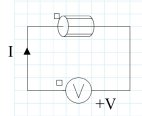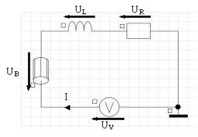# Examples of coils in 2D

## Introduction

We will model a coil supplied by a voltage source.

Two examples are shown:

• a circular coil modeled in an axisymmetric finite element domain
• a rectangular coil modeled in a planar finite element domain

## Modeling of a circular coil (axisymmetric domain)

The circular coil is represented:

• in the 2D axisymmetric finite elements domain by a region of stranded conductor type
• in the electrical circuit by a component of stranded conductor type

The different diagrams (real device, finite elements domain and electrical circuit) are represented in the figure below.

Real device 2D axisymmetric finite elements domain Electrical circuit## Modeling of a rectangular coil (plane domain)

The rectangular coil is represented:

• in the 2D plane finite elements domain by two regions: one for the in section of the coil, the other for the out section.
• in the electrical circuit by only one component of stranded conductor type

The different diagrams (real device, finite elements domain and electrical circuit) are given in the figure below.

In the electrical circuit, the resistance R and the inductance L correspond to the front and back parts of the conductor (end turns, …) that are not modeled in the finite elements domain.2D plane finite elements domainElectrical circuit: UV = UB + UR + UL
Note: The two regions of the type stranded conductors must be oriented in opposite directions in order that the currents in the in and out finite elements regions be opposed.